Smartick is an online platform for children to master math in only 15 minutes a day

Oct25

# Learn about Fractions: Halves, Thirds and Fourths

Welcome to Smartick’s blog! Today, we are going to talk about the fractions that we use on a daily basis.

I’m sure that you’ve heard of these expressions, and others like them on more than one occasion:

### Fractions

“I have half of a sandwich…”“You’ve eaten a third of your chocolate!”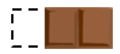“Could you cut me a fourth of this cheese, please?”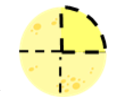“You need a half cup of water for this recipe”“The movie won’t start for a quarter of an hour”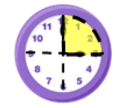But… what does a half, third or a fourth of a quantity mean? How do we calculate them?

We’re going to find out!

The expressions “a half” or “one half”, “a third” or “one third”, “a fourth” or “one fourth”, are the fractions that we most often use in everyday life. We use them when we refer to something that is divided into equal parts, or something that could be divided into equal parts.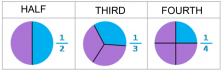If the whole is divided into two equal parts, each part is a HALF. A half is obtained by dividing a whole (1) by 2.

If the whole is divided into three equal parts, each part is a THIRD. A third is obtained by dividing a whole (1) by 3.

If a whole is divided into four equal parts, each part is a FOURTH. A fourth is obtained by dividing a whole (1) by 4.

### For Example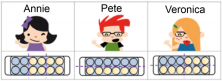“Annie, Veronica, and Pete went to the supermarket yesterday. Each one bought a dozen eggs because they were on sale. When they got home, Annie made an omelet using a third of her dozen eggs. Pete made scrambled eggs with half of his dozen, and Veronica used a fourth of her dozen to make a casserole. How many eggs did each person use?”

Starting off, we need to remember that a dozen eggs is 12 eggs. Now, let’s look at the information we are given:

• Annie used a third of her dozen of eggs. If we look at the picture, we can see that the dozen is divided into three equal parts and that she used one of these parts, or in other words, 4 eggs. We can calculate that by dividing: 12/3 = 4.
• Pete used a half dozen eggs. By looking at the picture, we can see the dozen is divided into two equal parts and that he used one of these parts, that is to say, 6 eggs. We can calculate this by dividing 12/2 = 6.
• Veronica used a fourth of her dozen of eggs. If we look at the picture, we see that the dozen is divided into four equal parts and that she used one of these parts, or in other words, 3 eggs. We can calculate this by dividing 12/4 = 3.